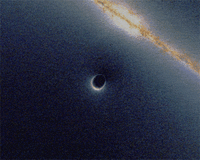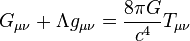# Gravitational singularity facts for kids

Kids Encyclopedia FactsLensing by a black hole. Animated simulation of gravitational lensing caused by a Schwarzschild black hole going past a background galaxy.
General relativity$G_{\mu \nu} + \Lambda g_{\mu \nu}= {8\pi G\over c^4} T_{\mu \nu}$
Einstein field equations
Phenomena
Kepler problem · Lenses · Waves
Frame-dragging · Geodetic effect
Event horizon · Singularity
Black hole

A gravitational singularity (sometimes called a spacetime singularity) is a term used to describe the center of a black hole where gravity is thought to approach infinity.

In the center of each black hole is a singularity, a point where infinite density develops as spacetime approaches it. Spacetime goes toward infinite curvature and matter is crushed to infinite density under the pull of infinite gravity. At a singularity, space and time cease to exist as we know them and current laws of physics cannot be applied to this region.

## Images for kidsGravitational singularity Facts for Kids. Kiddle Encyclopedia.